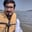Related Tags

python
numpy
array
communitycreator

# What is argsort() in NumPy?Arslan Bajwa

In Python, the NumPy library has a function called argsort(), which computes the indirect sorting of an array. It returns an array of indices along the given axis of the same shape as the input array, in sorted order.

## Syntax

numpy.argsort(array, axis, kind, order)

## Parameters

• array (array_like): This specifies the array we want to sort.

• axis (int or None), optional: This specifies the axis along which we want to sort. The default value is -1 specifying the last used axis. To use a flattened array, None is used.

• kind (quicksort, mergesort, heapsort, stable), optional: This specifies the sorting algorithm to be used. The default value is “quicksort”.

• order (str or list of str), optional: This specifies the order in which to compare fields.

## Return value

• ndarray, int: This returns indices of sorted array according to the specified parameters.

## Code

#importing numpy libarary
import numpy as np

#initializing input numpy array
array1 = np.array([[ 4, 6, 2], [ 5, 1, 3]])

#computing merge sort
result1 = np.argsort(array1, kind ='mergesort', axis = 0)
print ("merge sort indices", result1)

#computing heapsort
result2 = np.argsort(array1, kind ='heapsort', axis = 1)
print ("heap sort indices", result2)

#computing quicksort
result3 = np.argsort(array1, kind ='quicksort', axis = 1)
print ("quick sort indices", result3)

RELATED TAGS

python
numpy
array
communitycreator

CONTRIBUTORArslan Bajwa
RELATED COURSES

View all Courses

Keep Exploring

Learn in-demand tech skills in half the time